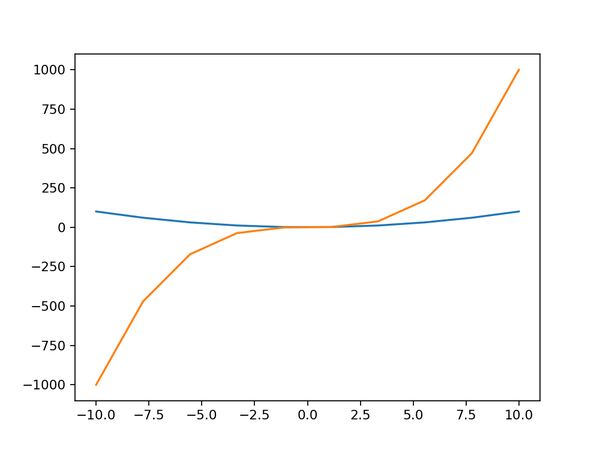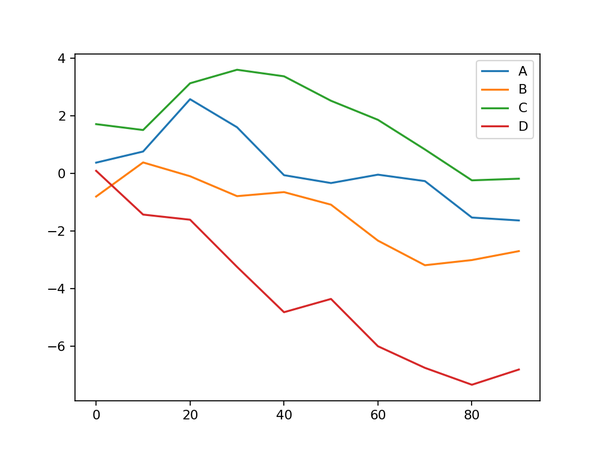# 线型图

Matplotlib为我们提供了大量的绘图种类，我们不可能一一介绍，学习量太大，也没必要。正确的方式是以典型绘图为例，掌握基本绘制技巧，然后在需要的时候，查阅官方文档，模仿操作。

```%matplotlib notebook
import numpy as np
import matplotlib as mpl
import matplotlib.pyplot as plt
```

```x = np.linspace(-10,10,200)
plt.plot(x, x**2)
plt.plot(x, x**3)
```plot方法的核心是plot(x,y)，x表示横坐标值的序列，y表示x某个坐标对应的y值，实际上就是y=f(x)函数。当只提供y的时候，x默认使用0-n的整数序列。这里的序列必然是个有限的点集，而不是我们想象中的无穷个点组成一条线。如果你的点很稀疏，那么图形看起来就像折线，如果点很多，看起来就比较圆滑，形似曲线。

```import pandas as pd
df = pd.DataFrame(np.random.randn(10, 4).cumsum(0),
columns=['A', 'B', 'C', 'D'],
index=np.arange(0, 100, 10))
df.plot()
```pandas和matplotlib的plot方法你愿意用哪个都行，但要注意参数格式和使用场景。